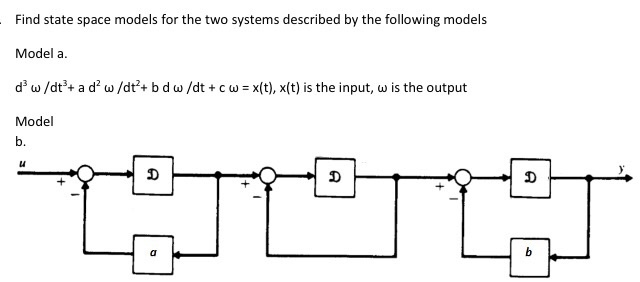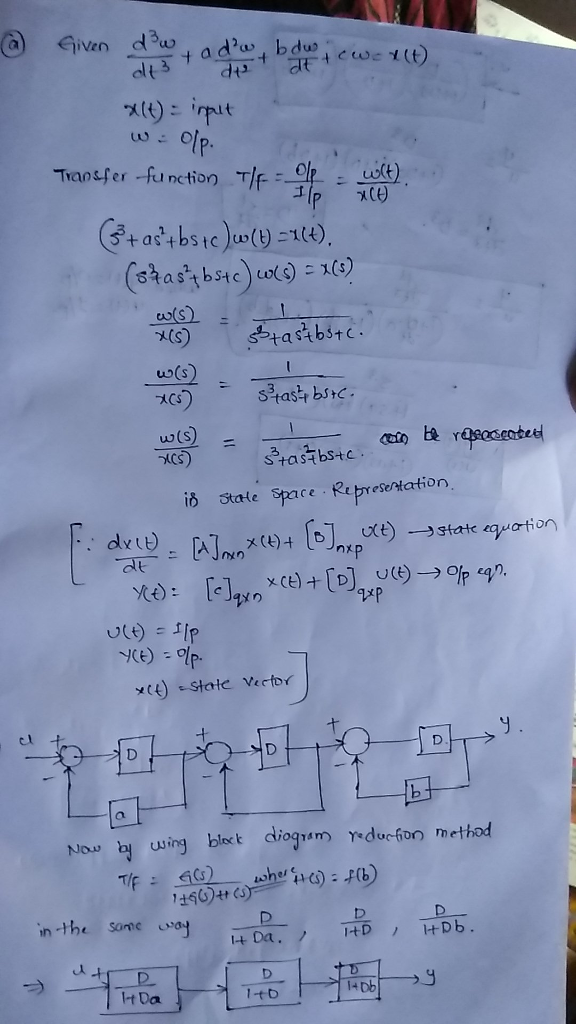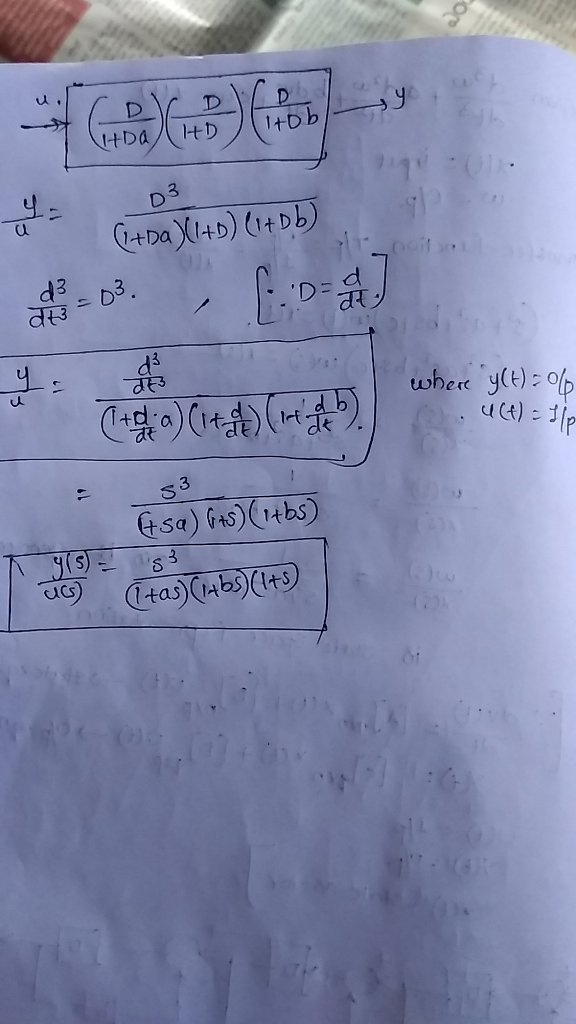# Homework Solution: Find state space models for the two systems described by the following models Model a. d^3 omega/dt^3 + a d^2 ome…Find state space models for the two systems described by the following models Model a. d^3 omega/dt^3 + a d^2 omega/dt^2 + b d omega/dt + c omega = x(t), x(t) is the input, omega is the output Model b.